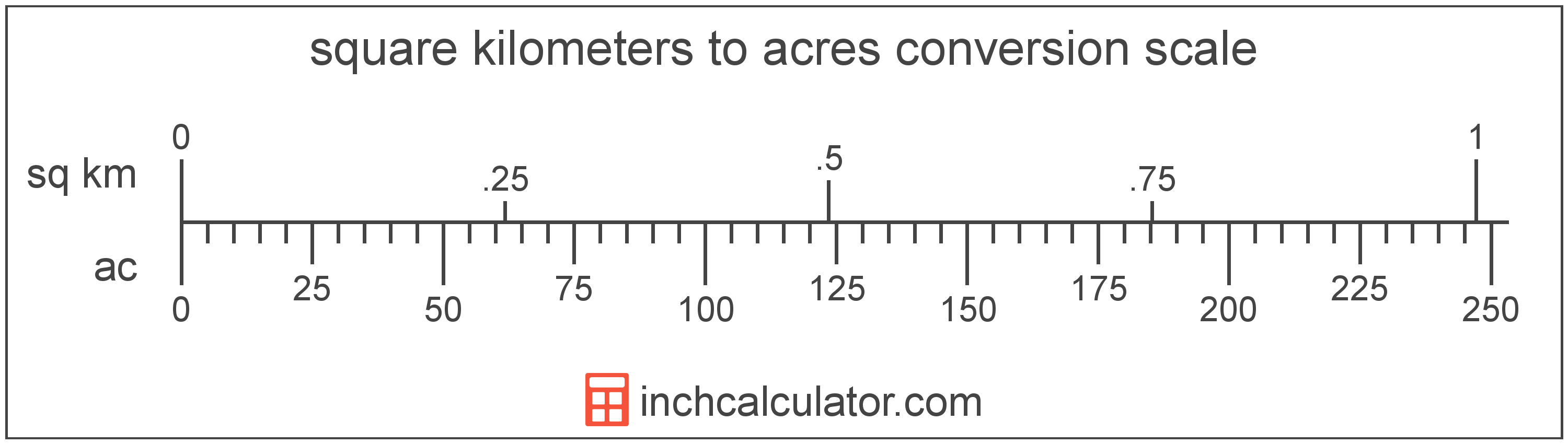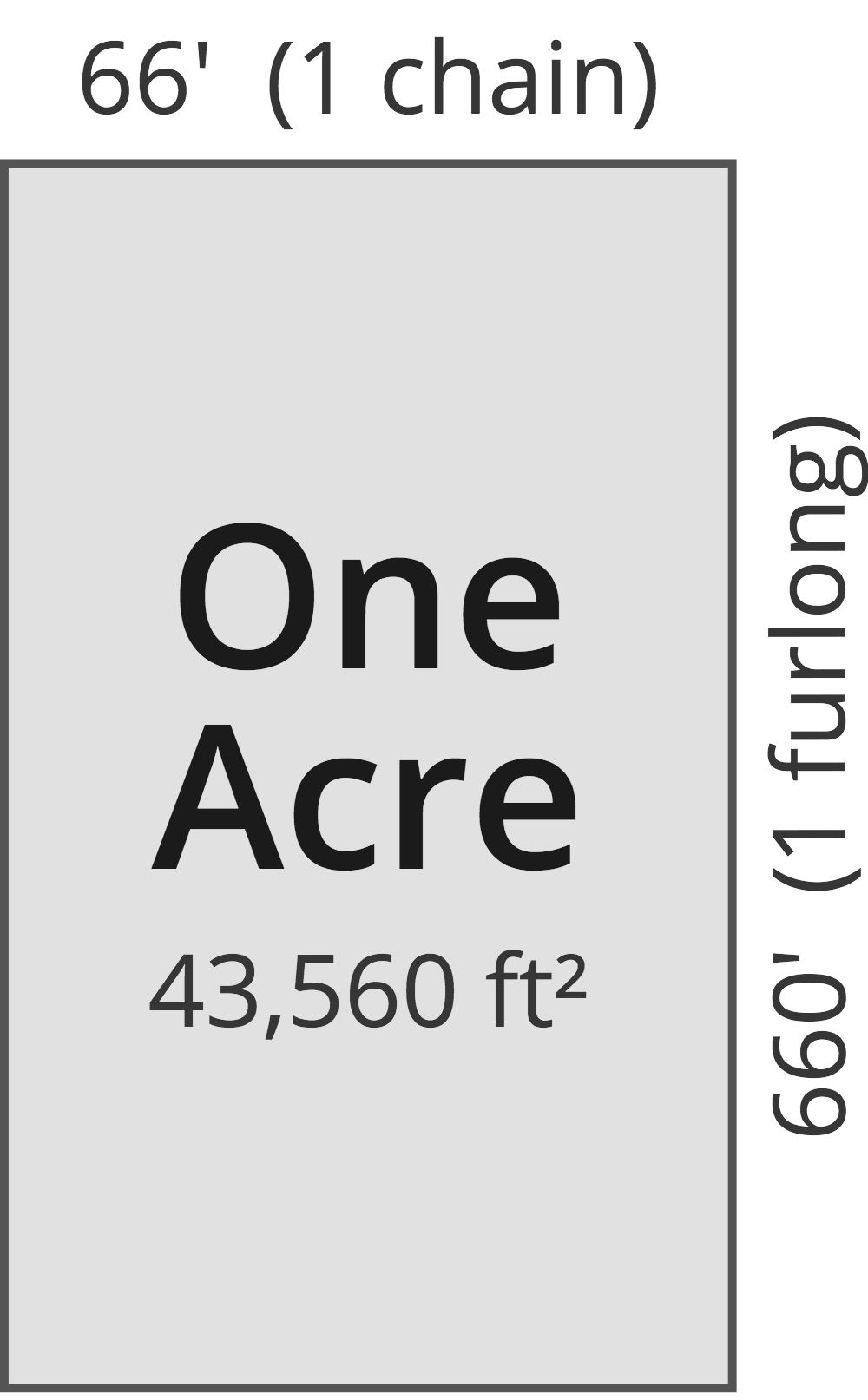# Square Kilometers to Acres Converter

Enter the area in square kilometers below to get the value converted to acres.

Results in Acres:1 sq km = 247.105381 ac

Do you want to convert acres to square kilometers?

## How to Convert Square Kilometers to Acres

To convert a measurement in square kilometers to a measurement in acres, multiply the area by the following conversion ratio: 247.105381 acres/square kilometer.

Since one square kilometer is equal to 247.105381 acres, you can use this simple formula to convert:

acres = square kilometers × 247.105381

The area in acres is equal to the area in square kilometers multiplied by 247.105381.

For example, here's how to convert 5 square kilometers to acres using the formula above.
acres = (5 sq km × 247.105381) = 1,235.526907 ac### How Many Acres Are in a Square Kilometer?

There are 247.105381 acres in a square kilometer, which is why we use this value in the formula above.

1 sq km = 247.105381 ac

## What Is a Square Kilometer?

One square kilometer is equivalent to the area of a square with sides that are each 1 kilometer in length. One square kilometer is equal to 0.386102 square miles or 1,000,000 square meters.

The square kilometer, or square kilometre, is a multiple of the square meter, which is the SI derived unit for area. In the metric system, "kilo" is the prefix for thousands, or 103. A square kilometer is sometimes also referred to as a square km. Square kilometers can be abbreviated as sq km, and are also sometimes abbreviated as km². For example, 1 square kilometer can be written as 1 sq km or 1 km².

## What Is an Acre?

One acre is defined as the area equal to a space that is one chain (66 ft) by one furlong (660 ft), or 10 square chains. That's equal to 43,560 square feet or 1/640 of a square mile for those unfamiliar with those units of measure.The acre is a US customary and imperial unit of area. Acres can be abbreviated as ac; for example, 1 acre can be written as 1 ac.

You can use an acreage calculator to measure the area of a plot of land in acres by locating the boundaries on a map.

## Square Kilometer to Acre Conversion Table

Table showing various square kilometer measurements converted to acres.
Square Kilometers Acres
1 sq km 247.11 ac
2 sq km 494.21 ac
3 sq km 741.32 ac
4 sq km 988.42 ac
5 sq km 1,236 ac
6 sq km 1,483 ac
7 sq km 1,730 ac
8 sq km 1,977 ac
9 sq km 2,224 ac
10 sq km 2,471 ac
11 sq km 2,718 ac
12 sq km 2,965 ac
13 sq km 3,212 ac
14 sq km 3,459 ac
15 sq km 3,707 ac
16 sq km 3,954 ac
17 sq km 4,201 ac
18 sq km 4,448 ac
19 sq km 4,695 ac
20 sq km 4,942 ac
21 sq km 5,189 ac
22 sq km 5,436 ac
23 sq km 5,683 ac
24 sq km 5,931 ac
25 sq km 6,178 ac
26 sq km 6,425 ac
27 sq km 6,672 ac
28 sq km 6,919 ac
29 sq km 7,166 ac
30 sq km 7,413 ac
31 sq km 7,660 ac
32 sq km 7,907 ac
33 sq km 8,154 ac
34 sq km 8,402 ac
35 sq km 8,649 ac
36 sq km 8,896 ac
37 sq km 9,143 ac
38 sq km 9,390 ac
39 sq km 9,637 ac
40 sq km 9,884 ac

## References

1. National Institute of Standards and Technology, Specifications, Tolerances, and Other Technical Requirements for Weighing and Measuring Devices, Handbook 44 - 2019 Edition, https://nvlpubs.nist.gov/nistpubs/hb/2019/NIST.HB.44-2019.pdf